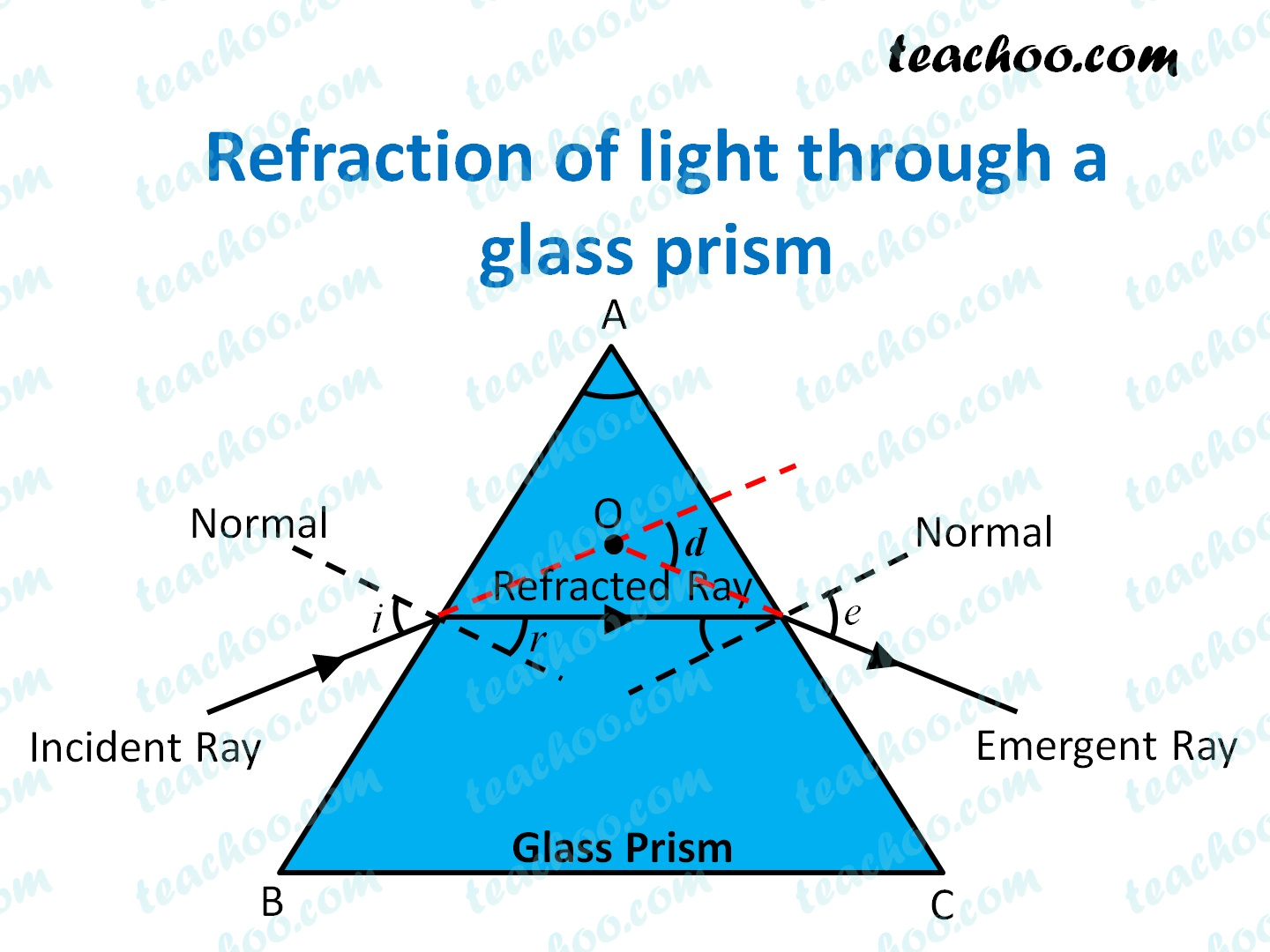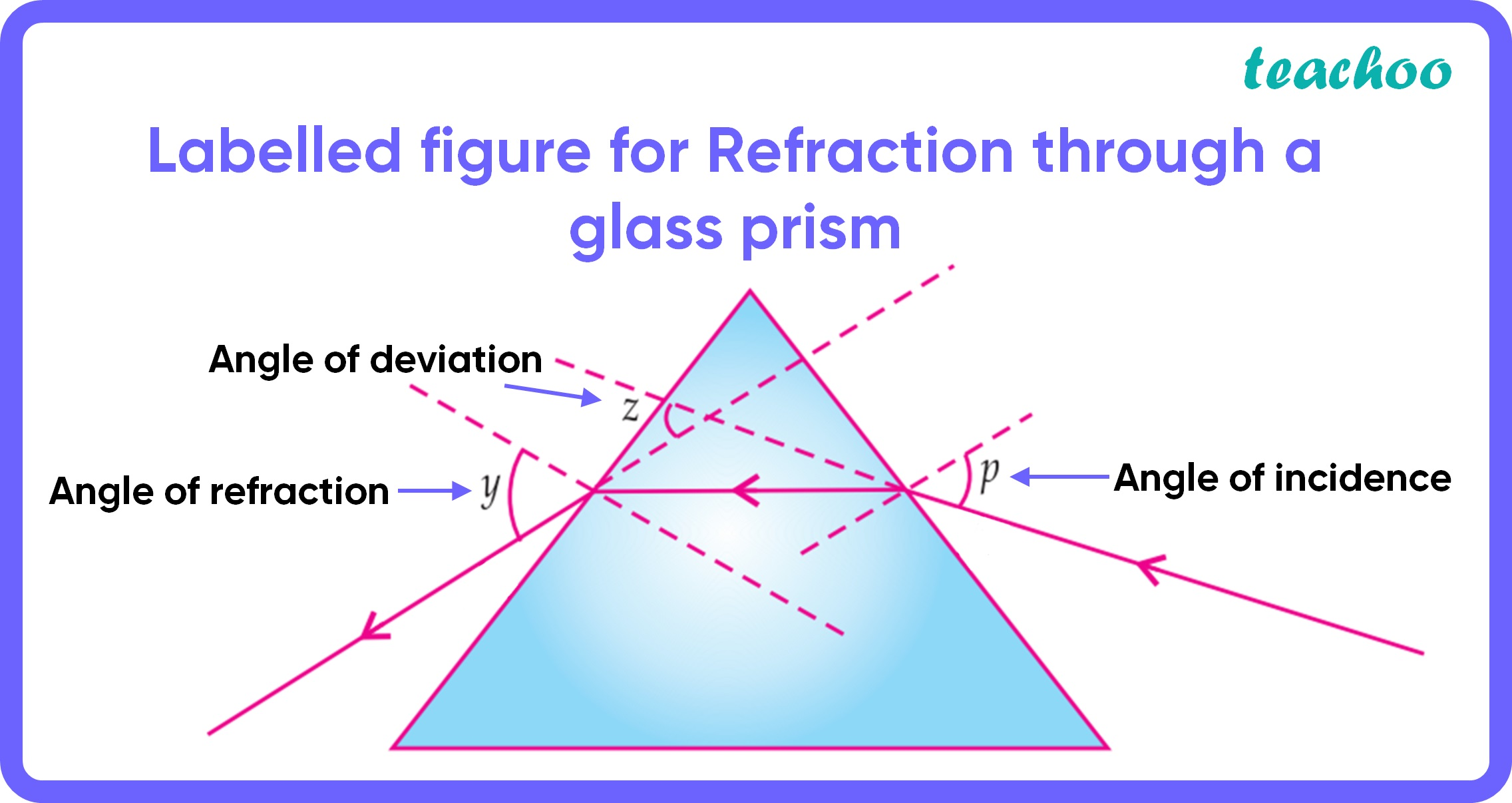MCQ from Past Year Papers

Class 10
Chapter 10 Class 10 - Light - Reflection and Refraction

## (d) p, z, y• Angle of incidence is the angle between incident ray and normal.
Here, incident ray is on the right side (check arrows).
So, here angle of incidence is angle p

• Angle of emergence is the angle between emergent ray and normal.
Here, emergent ray is on the left side (check arrows).
So, here angle of emergence is angle y

• Angle of deviation is the angle between emergent ray and incident ray (when produced backwards).
So, here angle of deviation is angle zSo, the correct answer is (c) - p, y, z

Get live Maths 1-on-1 Classs - Class 6 to 12